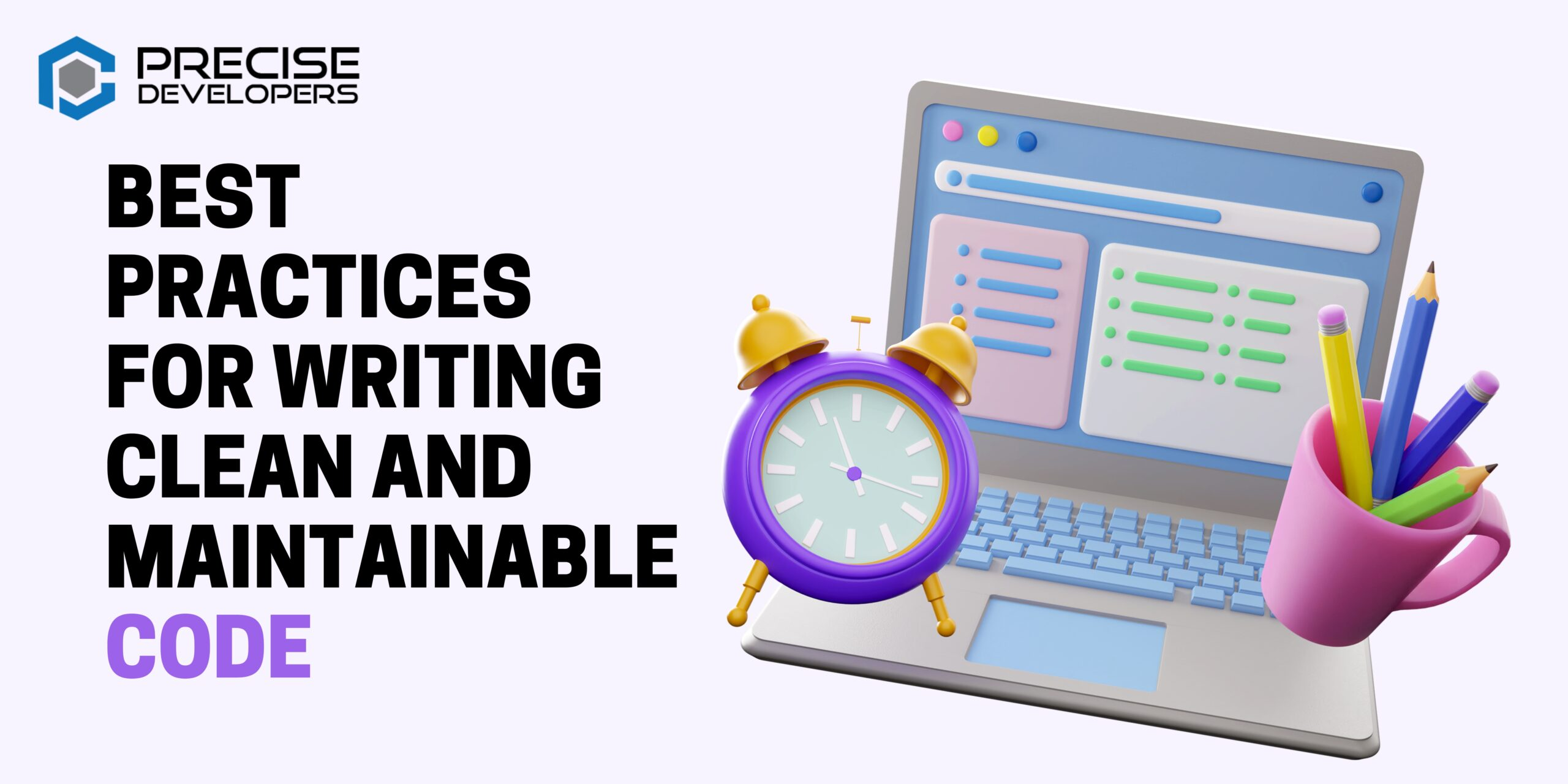We are 50+ professional Software Developers with more than 5+ years of experience in delivering solutions.

#### Contacts

+91 63548-55130 | +1 707-901-7072

Development# Best practices for writing clean and maintainable code

As a software developer, one of your primary goals should be to write code that is easy to understand, maintain, and modify. Not only does clean code make your own job easier, but it also helps other developers who may work on the same project in the future.

Here are some best practices to follow when writing clean and maintainable code:

Use descriptive and meaningful names for variables, functions, and other identifiers. This makes it easier to understand the purpose of each piece of code.

```// bad
let x = 10;
function y() {}

// good
let numStudents = 10;
function getAverageScore() {}```

Follow a consistent style guide. This can help ensure that your code is easy to read and follow and make it easier to integrate code written by other developers.

```<?php
// bad (mixing two different style guides)
function calculateSum(a, b) {
return a + b;
}

function calculateProduct(x, y) {
return x * y;
}

// good (following a single style guide)
function calculateSum(a, b) {
return a + b;
}

function calculateProduct(x, y) {
return x * y;
}```

Use comments to explain your code. While you should aim to write code that is self-explanatory, sometimes it is helpful to include a brief explanation of why you wrote certain code in a particular way.

```// calculate the average score for a list of students
function getAverageScore(students) {
let sum = 0;
for (let student of students) {
sum += student.score;
}
return sum / students.length;
}```

Break up your code into smaller, modular pieces. This makes it easier to understand and modify specific parts of your code.

```// bad (all code in a single function)
function processData(data) {
let cleanedData = cleanData(data);
let transformedData = transformData(cleanedData);
let analyzedData = analyzeData(transformedData);
let report = generateReport(analyzedData);
return report;
}

// good (code broken up into smaller, modular functions)
function processData(data) {
let cleanedData = cleanData(data);
let transformedData = transformData(cleanedData);
let analyzedData = analyzeData(transformedData);
let report = generateReport(analyzedData);
return report;
}

function cleanData(data) {
// code to clean data goes here
}

function transformData(data) {
// code to transform data goes here
}

function analyzeData(data) {
// code to analyze data goes here
}

function generateReport(data) {
// code to generate report goes here
}```

Write tests to ensure that your code is correct and remains correct after any changes are made.

```// test for calculateSum function
assert(calculateSum(1, 2) === 3);
assert(calculateSum(-1, 2) === 1);

// test for calculateProduct function
assert(calculateProduct(2, 3) === 6);
assert(calculateProduct(-2, 3) === -6);```

Use version control to track changes to your code. This can help you to revert to previous versions if necessary, as well as making it easier to collaborate with other developers.

```git init
git commit -m "initial commit"```

Document your code. In addition to writing comments within your code, it can be helpful to create separate documentation that explains the overall design and purpose of your code. This can make it easier for other developers to understand and work with your code.

```/**
* This function calculates the average score for a list of students.
*
* @param {Array} students - The list of students
* @return {number} The average score
*/
function getAverageScore(students) {
let sum = 0;
for (let student of students) {
sum += student.score;
}
return sum / students.length;
}
```

You may also like: Do you know MySQL has Blackhole?

In conclusion, writing clean and maintainable code is an important skill for any software developer. It helps to ensure that your code is easy to understand, modify, and maintain, which can save you time and effort in the long run. By following best practices such as using descriptive and meaningful names, following a consistent style guide, using comments to explain your code, breaking up your code into smaller, modular pieces, and writing tests to ensure correctness, you can write code that is of high quality and easy to work with. Remember to always strive to improve the readability and maintainability of your code, and it will pay off in the long run.

Our 5 Top Trending Blogs :